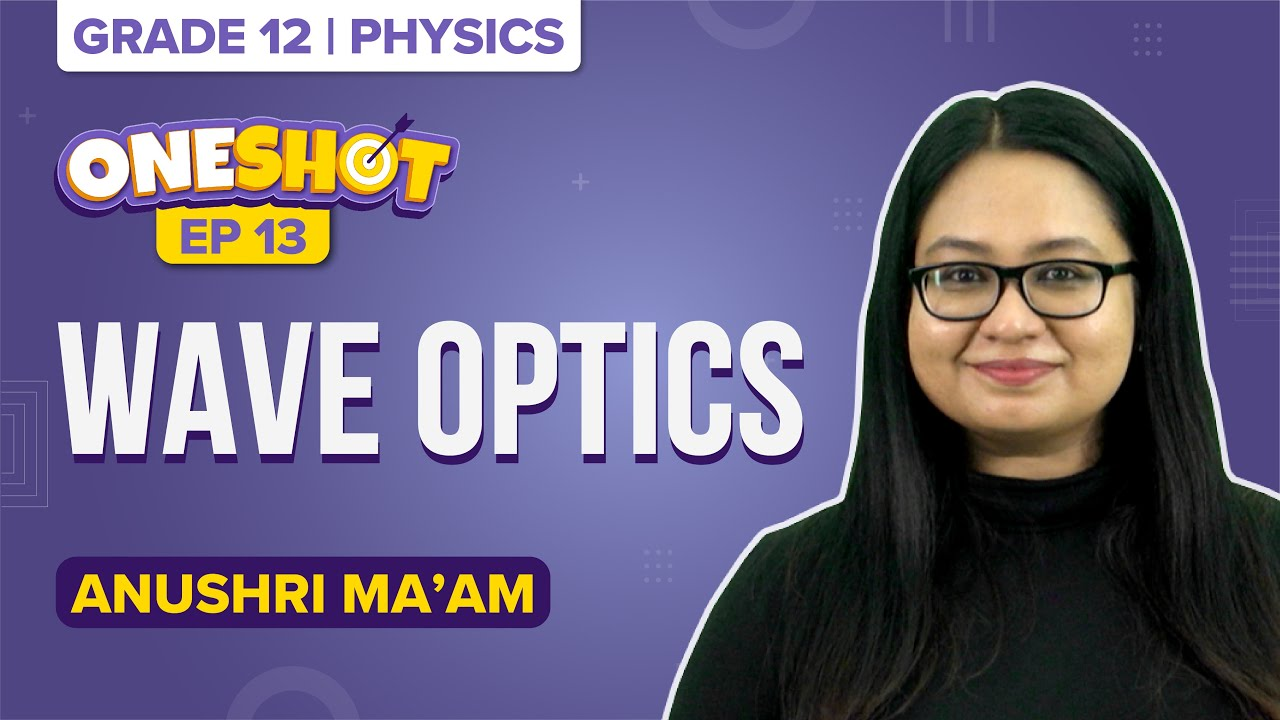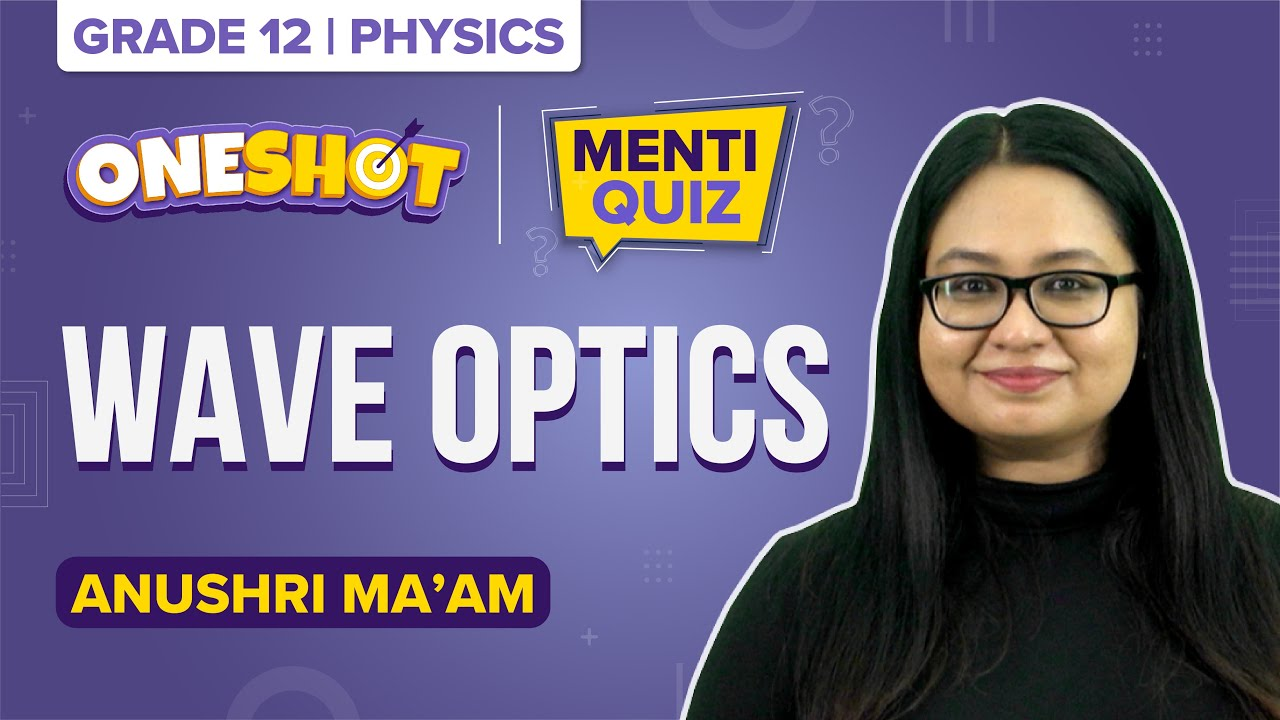# MCQs on Waves Optics for NEET

Wave Optics deals with the study of various phenomena such as polarization, diffraction, interference and other occurrences, which could not be explained using ray optics.

Q1: Polarisation phenomenon explains which nature of light?

1. Transverse
2. longitudinal
3. Both transverse and longitudinal
4. geometrical

Q2: A narrow slit is taken and a parallel beam of moving electrons is incident normally on it. At a larger distance from the slit, a fluorescent screen is placed. Which of the following statement is true if the size of the slit is further narrowed?

1. The diffraction pattern cannot be observed on the screen
2. The angular width of the central maxima of the diffraction pattern will increase
3. The angular width of the central maxima of the diffraction pattern will decrease
4. The angular width of the central maxima of the diffraction pattern remains the same

Answer: (c) The angular width of the central maxima of the diffraction pattern will decrease

Q3: How does the diffraction band of blue light look in comparison with the red light?

1. No changes
2. Diffraction pattern becomes narrower
4. Diffraction pattern disappears

Answer: (b) Diffraction pattern becomes narrower

Q4: Two coherent sources of light can be obtained from

1. Two different lamps
2. Two different lamps but of the same colour
3. Two different lamps of the same colour and having the same colour
4. None of these

Q5: Which of the following phenomenon is not explained by Huygen’s wave theory?

1. Diffraction
2. Interference
3. Polarisation
4. Photoelectric effect

Q6: What is the value of coherent time if L is the coherent length and c is the velocity of light?

1. cL
2. L/c
3. c/L
4. 1/Lc

Q7: The ratio of the amplitude of the two sources producing interference 3 : 5, the ratio of intensities at maxima and minima is

1. 25:6
2. 5:3
3. 16:1
4. 25:9

Q8: The colours on the soap bubble is due to

1. Interference
2. Polarisation
3. Diffraction
4. Reflection

Q9: Which of the following statements indicates that light waves are transverse?

1. Light waves can be polarised
2. Light waves can show interference
3. Light waves undergo diffraction
4. They travel in the vacuum

Answer: (a) Light waves can be polarised

Q10: In Young’s double-slit experiment, the phase difference between the light waves reaching the third bright fringe from the central fringe will be (λ=6000 Å)

1. Zero